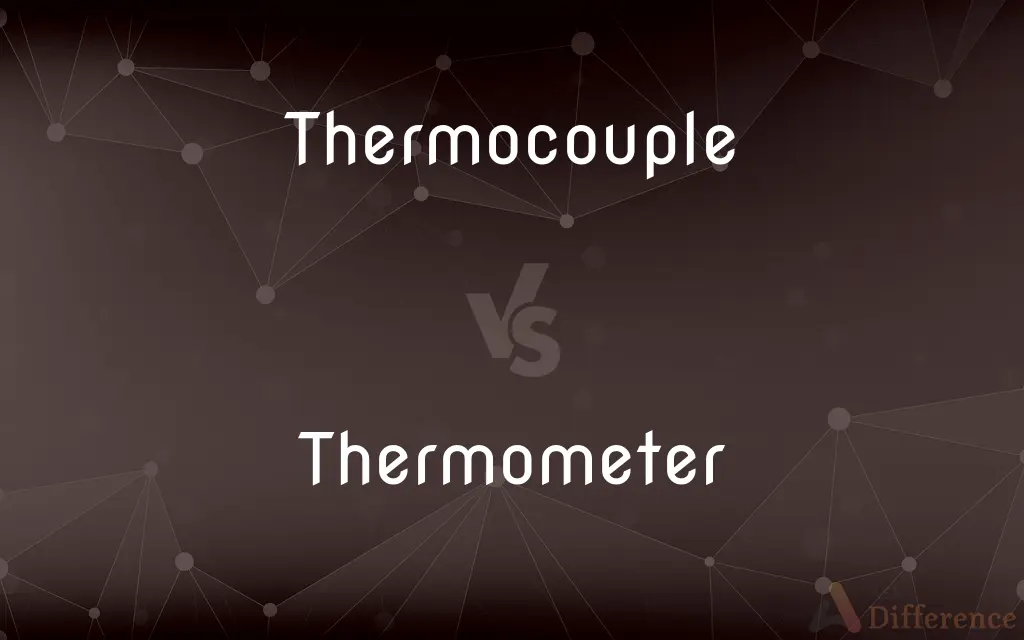# Thermocouple vs. Thermometer — What's the Difference?## Definitions

#### Thermocouple➦

A thermocouple is an electrical device consisting of two dissimilar electrical conductors forming an electrical junction. A thermocouple produces a temperature-dependent voltage as a result of Seebeck effect, and this voltage can be interpreted to measure temperature.

#### Thermometer➦

A thermometer is a device that measures temperature or a temperature gradient (the degree of hotness or coldness of an object). A thermometer has two important elements: (1) a temperature sensor (e.g.

#### Thermocouple➦

A thermoelectric device for measuring temperature, consisting of two wires of different metals connected at two points, a voltage being developed between the two junctions in proportion to the temperature difference.

#### Thermometer➦

An instrument for measuring temperature, especially one having a graduated glass tube with a bulb containing a liquid, typically mercury or colored alcohol, that expands and rises in the tube as the temperature increases.

#### Thermocouple➦

A thermoelectric device used to measure temperatures accurately, especially one consisting of two dissimilar metals joined so that a potential difference generated between the points of contact is a measure of the temperature difference between the points.

#### Thermometer➦

An apparatus used to measure temperature.

#### Thermocouple➦

(physics) A transducer consisting of two different metals welded together at each end; a voltage is produced that is proportional to the difference in temperature between the two junctions (one of which is normally held at a known temperature)

#### Thermometer➦

An instrument for measuring temperature, founded on the principle that changes of temperature in bodies are accompanied by proportional changes in their volumes or dimensions.

#### Thermocouple➦

A thermoelectric couple.

#### Thermometer➦

Measuring instrument for measuring temperature

#### Thermocouple➦

A kind of thermometer consisting of two wires of different metals that are joined at both ends; one junction is at the temperature to be measured and the other is held at a fixed lower temperature; the current generated in the circuit is proportional to the temperature difference